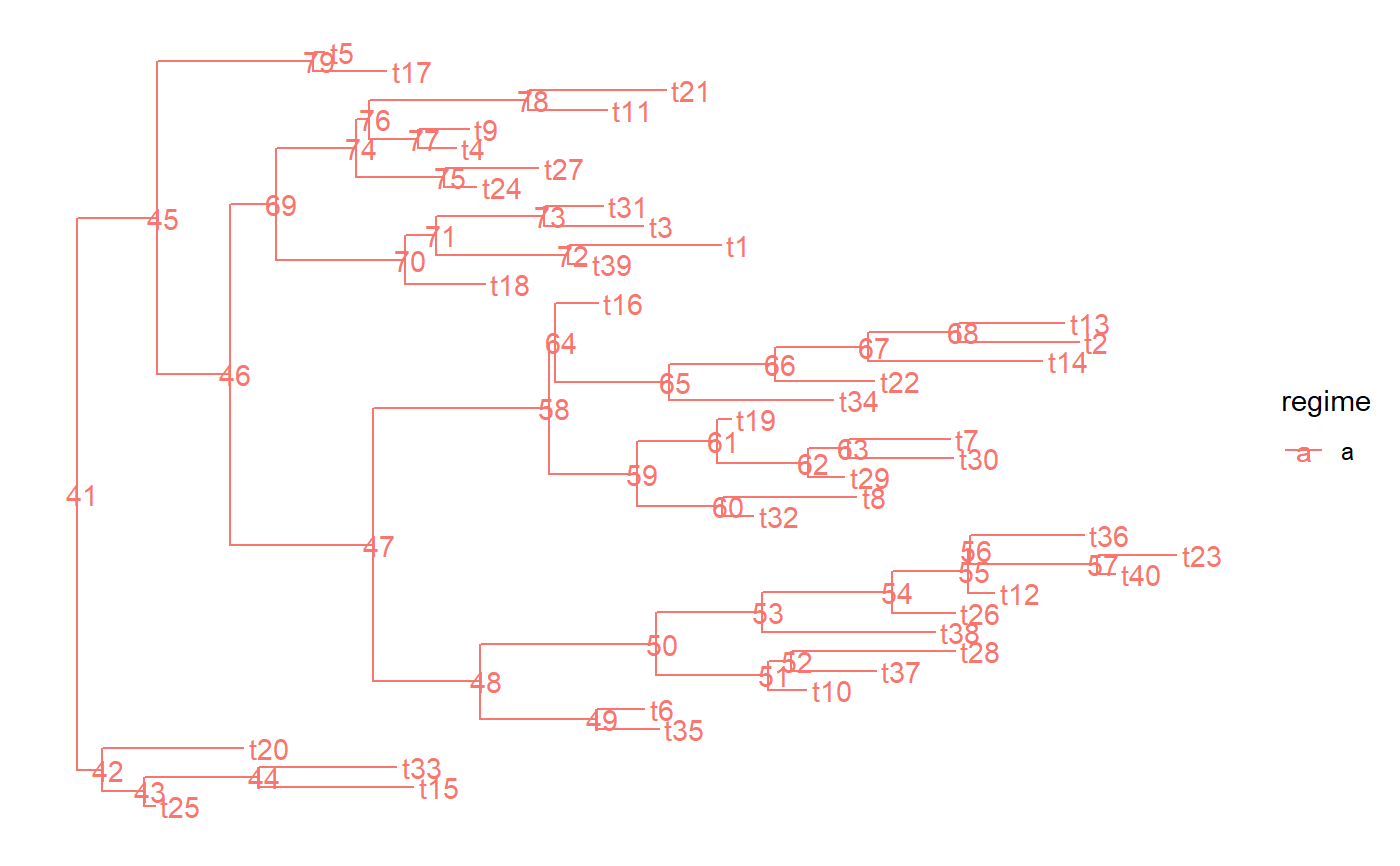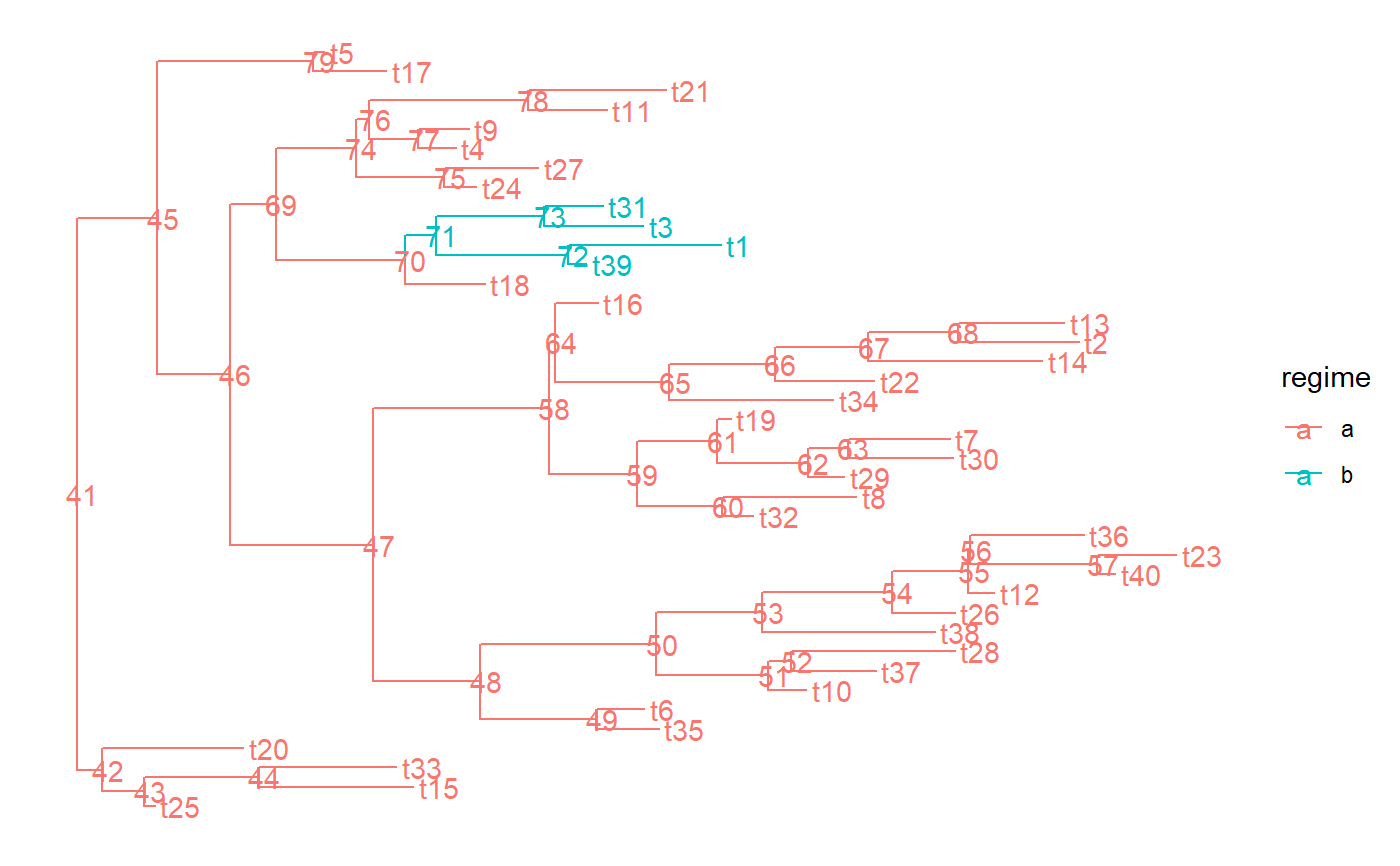Set regimes for the parts in a tree

PCMTreeSetPartRegimes(tree, part.regime, setPartition = FALSE, inplace = TRUE)

## Arguments

tree a PCMTree object. a named vector containing regimes to be assigned to some of or to each of the parts in the tree. a logical indicating if the partition of the tree should be set as well. If this argument is set to TRUE, the names of part.regime are passed as the nodes argument in a call to PCMTreeSetPartition. Default: FALSE. a logical indicating if the change should be done to the tree in the calling environment (TRUE) or a copy of the tree with set edge.part member should be returned (FALSE). Default is TRUE.

## Value

If inplace is TRUE nothing, otherwise a copy of the tree with set edge.part and part.regime members.

PCMTree

## Examples

tree <- PCMTree(ape::rtree(25))
PCMTreeGetPartition(tree)#> 26
#> 26 PCMTreeGetPartRegimes(tree)#> 26
#>  1 PCMTreeGetPartNames(tree)#>  "26"
PCMTreeSetPartRegimes(tree, c(26 = 2))
PCMTreeGetPartition(tree)#> 26
#> 26 PCMTreeGetPartRegimes(tree)#> 26
#>  2 PCMTreeGetPartNames(tree)#>  "26"
PCMTreeSetPartRegimes(tree, c(26 = "global-regime"))
PCMTreeGetPartition(tree)#> 26
#> 26 PCMTreeGetPartRegimes(tree)#>              26
#> "global-regime" PCMTreeGetPartNames(tree)#>  "26"
# This should fail because no partition with nodes 26, 28 and 41 has been
# done.
ggplot2::should_stop(
PCMTreeSetPartRegimes(tree, c(26 = "a", 28 = "b", 41 = "c")))
# This should succeed and change the partition as well as regime assignment
PCMTreeSetPartRegimes(
tree, c(26 = "a", 28 = "b", 41 = "c"), setPartition = TRUE)
PCMTreeGetPartition(tree)#> 26 28 41
#> 26 28 41 PCMTreeGetPartRegimes(tree)#>  26  28  41
#> "a" "b" "c" PCMTreeGetPartNames(tree)#>  "26" "28" "41"

set.seed(1, kind = "Mersenne-Twister", normal.kind = "Inversion")
# number of tips
N <- 40

# tree with one regime
tree.a <- ape::rtree(N)

tree.a <- PCMTree(tree.a)

PCMTreeSetPartRegimes(
tree.a,
part.regime = structure("a", names = as.character(N+1L)),
setPartition = TRUE,
inplace = TRUE)

# \donttest{
PCMTreePlot(tree.a) + ggtree::geom_nodelab() + ggtree::geom_tiplab()# }
tree.ab <- tree.a
PCMTreeSetPartRegimes(
tree.ab,
part.regime = structure(c("a", "b"), names = as.character(c(N+1L, N+31L))),
setPartition = TRUE,
inplace = TRUE)
# \donttest{
PCMTreePlot(tree.ab) + ggtree::geom_nodelab() + ggtree::geom_tiplab()# }